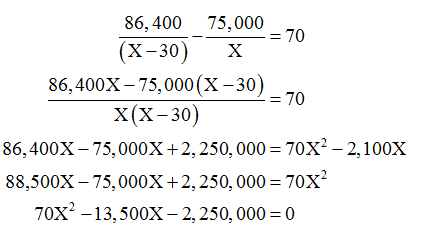# A retailer bought several pairs of jeans for \$75,000.He sold all but 30 pairs for \$86,400 making a profit of \$70 on each pair sold.How many pairs of jeans did the retailer buy?

Question
4 views

A retailer bought several pairs of jeans for \$75,000.He sold all but 30 pairs for \$86,400 making a profit of \$70 on each pair sold.How many pairs of jeans did the retailer buy?

check_circle

Step 1

Let the number of pairs bought by the retailer be X numbers. The cost of jeans brought by the retailer is \$75,000 whereas 30 pairs were sold for \$86,400.it is also given that the profit that the individual made on each unit he sells is \$70. Thus, the equation can be written as follows:Step 2

The quadratic equation we received above can be solved in order to calculate the number of pairs that the retailer bought. For this purpose, the value of a will be 70, b will be -13,500 and the value of c will be -2,250,000. The equation can be resolv...

### Want to see the full answer?

See Solution

#### Want to see this answer and more?

Solutions are written by subject experts who are available 24/7. Questions are typically answered within 1 hour.*

See Solution
*Response times may vary by subject and question.
Tagged in